# Java - Math Class

## Java - Math Class

The java math class delegates advanced mathematical calculations than the basic java math operators. Below are its properties:

• Present in the java.lang package.
• Holds methods for finding
• the maximum or minimum of two values,
•  rounding values,
• logarithmic functions,
• square root, and
• trigonometric functions
• Constants present in the java.lang.math package:
• E - A double value which is closer to the base value of natural logarithms
• PI - A double value which is closer to the ratio of the circumference of a circle to its diameter.
• Contains following methods:

#### Basic Mathematical Methods Explanation

 Methods (Basic Mathematical Methods) Explanation double abs(double d),float abs(float f),int abs(int i),long abs(long lng) Calculates the smallest integer that is greater than or equal to the argument. Returned as a double. double floor(double d) Calculates the largest integer that is less than or equal to the argument. Returned as a double. double floorDiv(double d, double e) divides one integer (int or long) by another, and rounds the result down to the nearest integer value. double rint(double d) Calculates the integer that is closest in value to the argument. Returned as a double. long round(double d),int round(float f) Calculates the closest long or int, as indicated by the method's return type, to the argument. double min(double arg1, double arg2),float min(float arg1, float arg2),int min(int arg1, int arg2),long min(long arg1, long arg2) Calculates the smaller of the two arguments. double max(double arg1, double arg2),float max(float arg1, float arg2),int max(int arg1, int arg2),long max(long arg1, long arg2) Calculates the larger of the two arguments. double round(double d) & double random() round : rounds a float or double to the nearest integer using normal math round rules. random : Calculates a random floating point number between 0 and 1. double ceil(double d) Calculates the smallest integer that is greater than or equal to the argument. Returned as a double.

#### Exponential and Logarithmic Methods Explanation :

 Methods (Exponential and Logarithmic Methods) Explanation double exp(double d) Calculates the base of the natural logarithms, e, to the power of the argument. double log(double d) Calculates the natural logarithm of the argument. double log10(double d) Calculates the natural logarithm of the argument using 10 as the base. double pow(double base, double exponent) Calculates the value of the first argument raised to the power of the second argument. double sqrt(double d) Calculates the square root of the argument. double sin(double d) Calculates the sine of the specified double value. double cos(double d) Calculates the cosine of the specified double value. double tan(double d) Calculates the tangent of the specified double value. double asin(double d) Calculates the arcsine of the specified double value. double acos(double d) Calculates the arccosine of the specified double value. double atan(double d) Calculates the arctangent of the specified double value. double atan2(double y, double x) Converts rectangular coordinates (x, y) to polar coordinate (r, theta) and calculates theta. double sinh(double d) calculates the hyperbolic sine value of a value between 1 and -1 double cosh(double d) calculates the hyperbolic cosine value of a value between 1 and -1 double tan(double d) calculates the hyperbolic tangens value of a value between 1 and -1 double toDegrees(double d) Converts the argument to degrees. double toRadians(double d) Converts the argument to radians.

#### Sample Code :

`public class MyMathSample {    public static void main(String[] args)     {        double a = -200.12, b = 20.94, c = 3.67;        int d = 15, e = 30;		double degrs = 45.0;        double rdns = Math.toRadians(degrs);		System.out.println("\n\nWikiTechy - Demo on Java.Lang.Math Package\n");          System.out.printf("The absolute value " + "of %.3f is %.3f%n",                           a, Math.abs(a));        System.out.printf("The ceiling of " + "%.2f is %.0f%n",                           b, Math.ceil(b));        System.out.printf("The floor of " + "%.2f is %.0f%n",                           b, Math.floor(b));        System.out.printf("The rint of %.2f " + "is %.0f%n",                           b, Math.rint(b));        System.out.printf("The max of %d and " + "%d is %d%n",                          d, e, Math.max(d, e));        System.out.printf("The min of of %d " + "and %d is %d%n",                          d, e, Math.min(d, e));        System.out.printf("exp(%.3f) " + "is %.3f%n",                          b, Math.exp(b));        System.out.printf("log(%.3f) is " + "%.3f%n",                          b, Math.log(b));        System.out.printf("pow(%.3f, %.3f) " + "is %.3f%n",                          b, c, Math.pow(b, c));        System.out.printf("sqrt(%.3f) is " + "%.3f%n",                          b, Math.sqrt(b));	 	System.out.format("The sine of %.1f " + "degrees is %.4f%n",                          degrs, Math.sin(rdns));        System.out.format("The cosine of %.1f " + "degrees is %.4f%n",                          degrs, Math.cos(rdns));		System.out.format("The arcsine of %.4f " + "is %.4f degrees %n",                           Math.sin(rdns),                           Math.toDegrees(Math.asin(Math.sin(rdns))));    }}`

Code Explanation: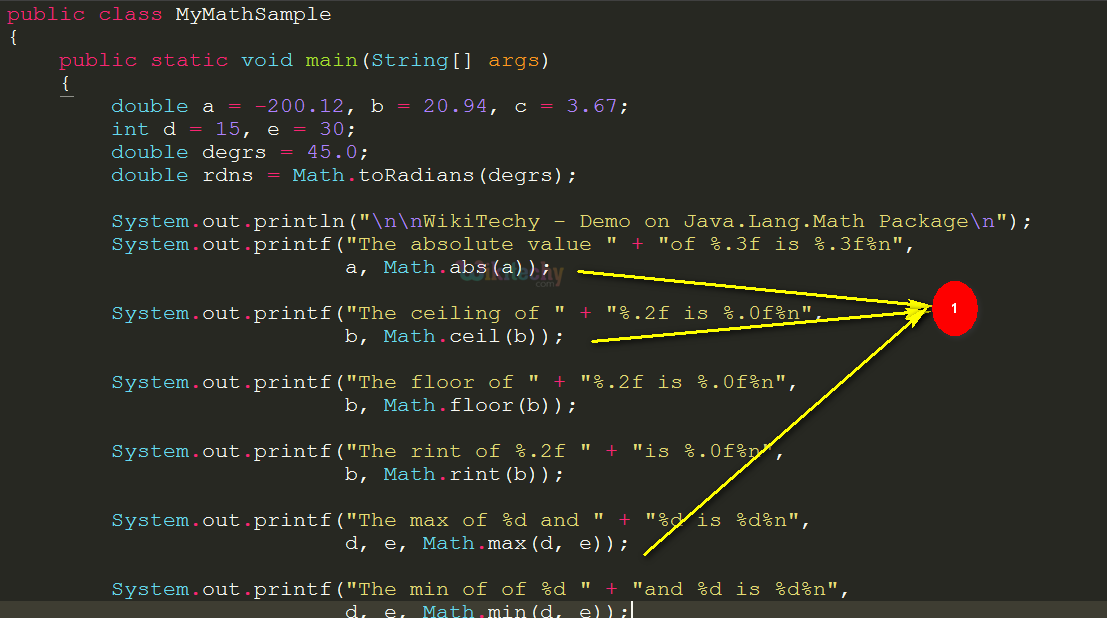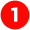Basic Math Class Methods are implemented in the code via the lines

• Math.abs(a));
• Math.ceil(b));
• Math.floor(b));
• Math.rint(b));
• Math.max(d, e));
• Math.min(d, e));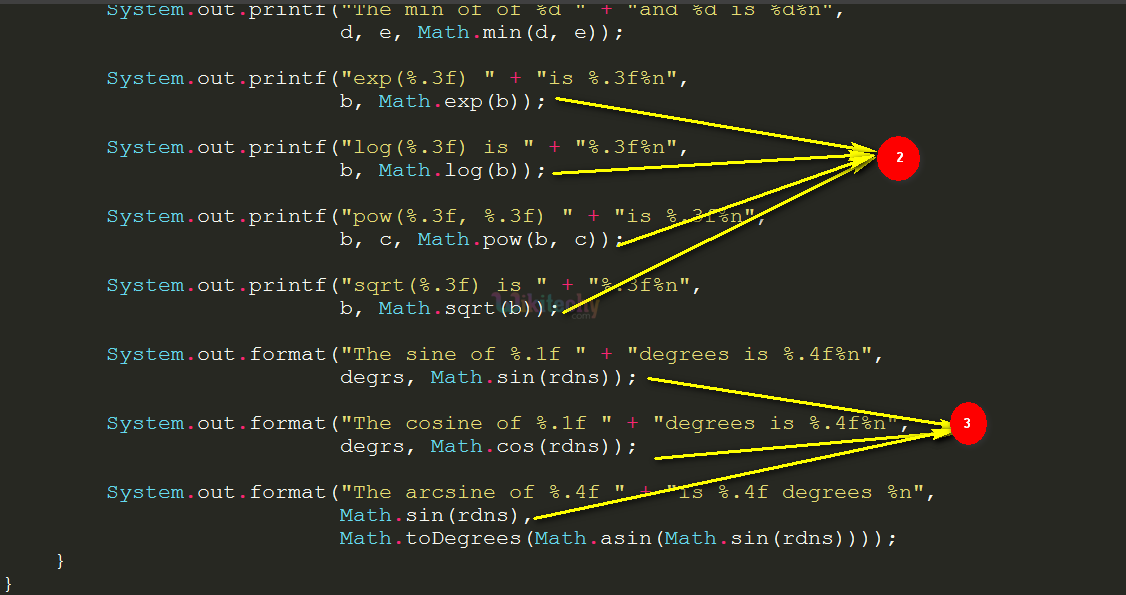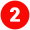Exponential and Logarithmic Methods are implemented via the code

• Math.exp(b));
• Math.log(b));
• Math.pow(b, c));
• Math.sqrt(b));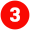Trigonometric Methods are depicted here using the code

• Math.sin(rdns));
• Math.cos(rdns));
• Math.toDegrees(Math.asin(Math.sin(rdns))));

## Sample Code: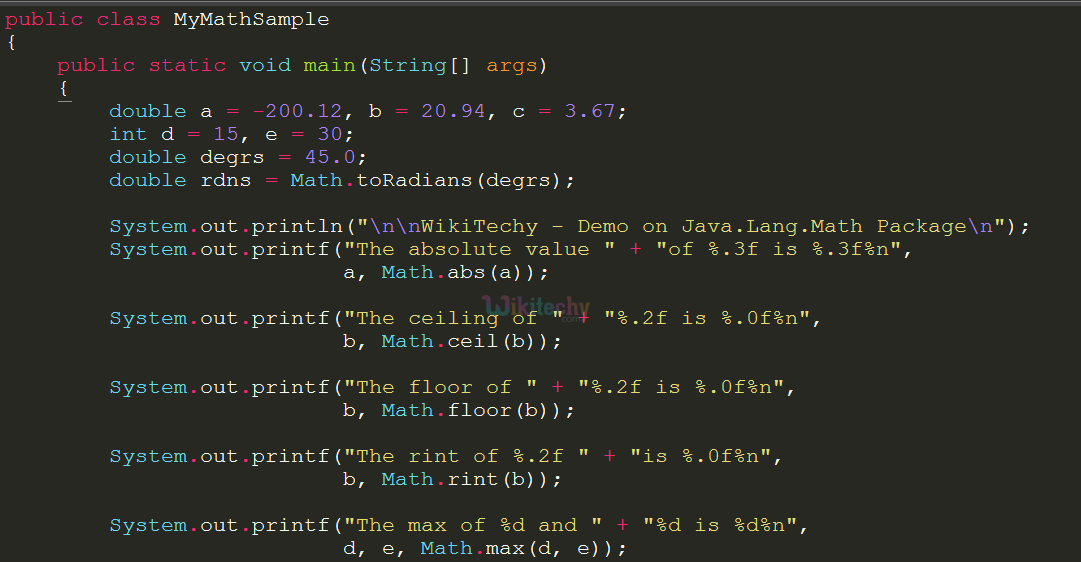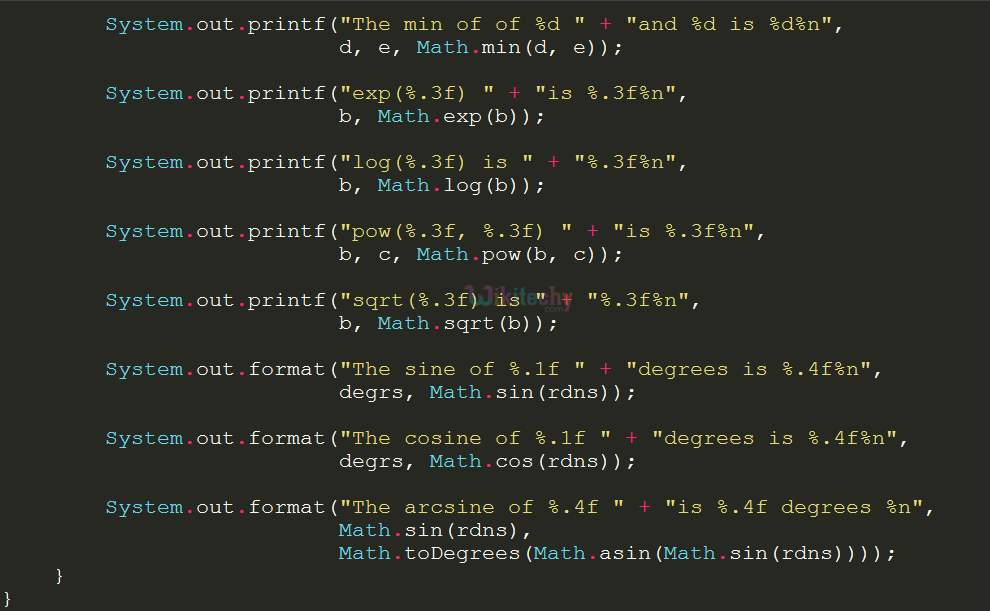## Output: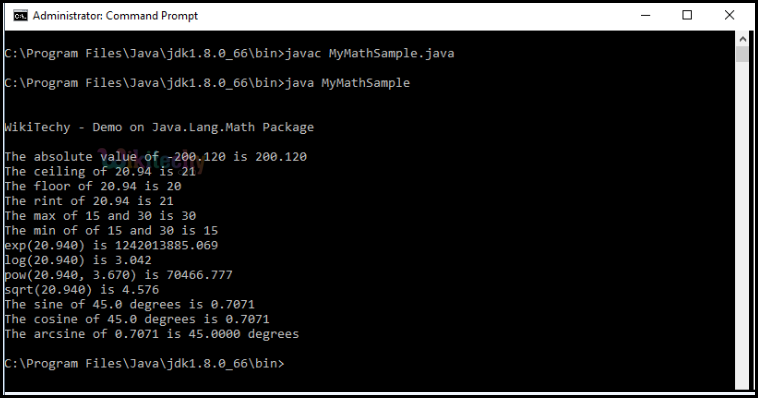Bug Bounty
Webinar